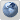SPCC - Computer Science Department

# Maths Workout##Maths Workout

Tip / Advice on large number multiplication!
You can achieve great results if you follow these simple guidelines. We will show you how visualize the problem and to multiply 24 and 25 in this example.
First, break down both numbers to the power of ten. Now you have 20+4 multiplied by 20+5.
Multiply each number by every other, and memorize each result: 20×20=400, 20×5=100, 4×20=80, 4×5=20.
Then you carefully add up those numbers; 400+100+80+20 gives 600. Was that difficult? Hopefully this will inspire you to math greatness.